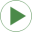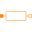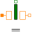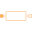# Type Information

Real Quantity: Reluctance Unit: H-1

# Used in Examples (5)EddyCurrentLosses Modelica.Magnetic.FundamentalWave.Examples.Components Comparison of equivalent circuits of eddy current loss modelsSinglePhaseInductance Modelica.Magnetic.FundamentalWave.Examples.Components Single phase inductanceMultiPhaseInductance Modelica.Magnetic.FundamentalWave.Examples.Components Multi phase inductanceMultiPhaseInductance Modelica.Magnetic.QuasiStatic.FundamentalWave.Examples.Components Multi phase inductanceEddyCurrentLosses Modelica.Magnetic.QuasiStatic.FundamentalWave.Examples.Components Comparison of equivalent circuits of eddy current loss models

# Used in Components (9)ConstantReluctance Modelica.Magnetic.FluxTubes.Basic Constant reluctanceLeakageWithCoefficient Modelica.Magnetic.FluxTubes.Basic Leakage reluctance with respect to the reluctance of a useful flux path (not for dynamic simulation of actuators)PartialFixedShape Modelica.Magnetic.FluxTubes.Interfaces Base class for flux tubes with fixed shape during simulation; linear or non-linear material characteristicsPartialForce Modelica.Magnetic.FluxTubes.Interfaces Base class for flux tubes with reluctance force generation; constant permeabilityPartialLeakage Modelica.Magnetic.FluxTubes.Interfaces Base class for leakage flux tubes with position-independent permeance and hence no force generation; mu_r=1ConstantReluctance Modelica.Magnetic.QuasiStatic.FluxTubes.Basic Constant reluctanceLeakageWithCoefficient Modelica.Magnetic.QuasiStatic.FluxTubes.Basic Leakage reluctance with respect to the reluctance of a useful flux path (not for dynamic simulation of actuators)PartialFixedShape Modelica.Magnetic.QuasiStatic.FluxTubes.Interfaces Base class for flux tubes with fixed shape during simulation; linear or non-linear material characteristicsPartialLeakage Modelica.Magnetic.QuasiStatic.FluxTubes.Interfaces Base class for leakage flux tubes with position-independent permeance and hence no force generation; mu_r=1